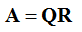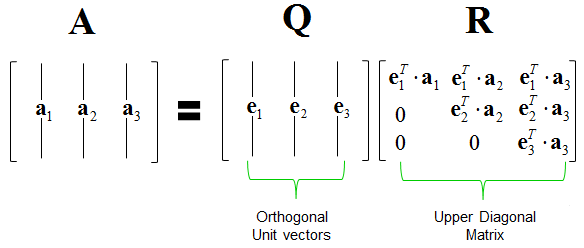Engineering Math - Quick Reference                                 Home : www.sharetechnote.com QR Decomposition   QR Decomposition is a method of splitting a matrix into multiplication of following two matrix.What does each of the matrix mean ? Each of the matrix in the equation indicates as shown below. As you see here, Q is made up of Orthogonal Unit Vectors and R is a Upper Triangular Matrix.How do you calculate this ? If you look at the contents of Q and R, you will notice.. once you get Q matrix R can be derivated mechanically from the column vectors of A and element and the column vectors of Q. So the only thing you have to consider is how to derive Q matrix. Q can be derived from A by a special method called Gram Schmidt Process. However, Gram Schmidt Process will derive an Orthogonal matrix but it is not guaranteed that all the vectors in the orthogonalized matrix. To make each of the vectors in the orthogonalized matrix, you just need to normalize each of the column vector of orthogonalized matrix produced by Gram Schmidt Process. Now do you know what do you have to do ?  Yes, study Gram Schmidt Process :)